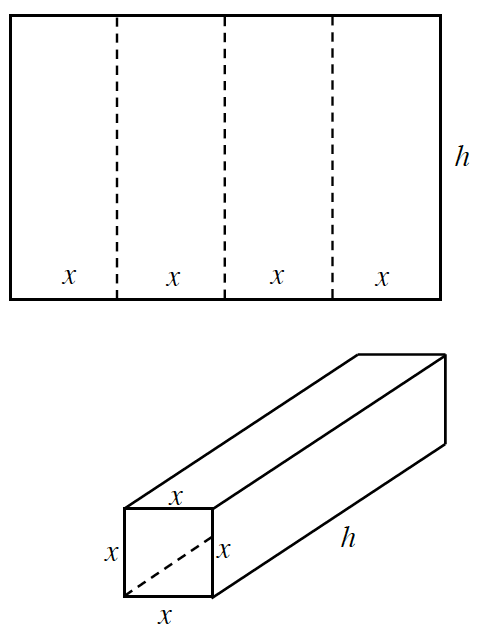### Home > PC3 > Chapter 7 > Lesson 7.2.2 > Problem7-90

7-90.A rectangular piece of cardboard has a perimeter of $24$ feet. The cardboard is creased in such a way that it can be folded to form a square tube with open ends as shown in the diagram.

1. Write an equation for the volume of the box in terms of $h$ and $x$.

$8x+2h=24$

2. Now, write the volume of the box using only $x$.

$V=x^2h$

3. What is an appropriate domain for the function you wrote in part (b)?

Of course $x>0$. The 'largest' value of $x$ occurs when the height is approximately $0$. Use your equation from part (a) to determine the largest possible value of $x$.

4. Graph the volume function and locate the point that represents the maximum volume.

Use a graphing calculator to graph your equation from part (b).